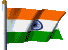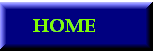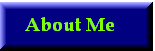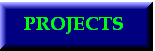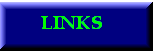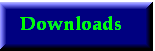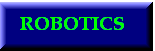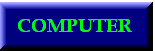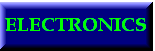CIRCUIT DIAGRAMBASICS AND SPECIFICATIONS
We all know that different IC's have different input and output pins.The first procedure is to program which pins are for input and output. This programming is done by writing data
into control register. That is the name of that register is control register. We can only input 13 data from parallel port at a time. But this is not sufficient. So what I am doing is that I am storing data into data register and read the output from all pins.

SOFTWARE:
Operating System: Windows'98(recommended),Windows XP(with Userport.zip installed)
also work in Windows'95 and ME
Compiler : Turbo C++ 3.0

source code

Programming Language: C(with graphics)
IC:
This will work with all digital IC's work on 5v. I tested it for 74/40/45
series.
COST:
Cost is around Rs.300 depending on the price of IC's in your place
(don't forget to buy 74LS244 not 74HC244)
BIOS SETTINGS:
parallel port mode-[Bi-directional]
Interrupt -[IRQ7]
if ur Bios is not having these option set parallel port to a mode other than
ECP, EPP, EPP+ECP.

SOFTWARE'S USER INTERFACEFrom this window u will be selecting one IC then u will get the next window
given below.Before this there is hardware checking. If you want this
software mail me.WORKING:
Before reading this you have to see the datasheet of the IC's and how it work(if you don't know about these IC's). First we see the testing of combinationl circuits.So I am taking a simple IC
7404, same logic for all other IC's working without clock.Before programming you have to see the control port pins. You can see that c2,c1',c0' . So see the below table for corresponding IC's.From the table we can see that if you want the output of the decoder to be Q0 then we have to write 3 to control port ,i.e,011 so the output of port will be 000. Always use this table when you are seeing code.
decoder software
0 3
1 2
2 1
3 0
4 7
5 6
7 5
First connect pin1 of IC to the 1 shown in circuit diagram.Now next aim to set control register. You can see that pin 1,3,5 are for input and 2,4,6 for output and 7 for ground, 8 is not used in diagram. So we want to send voltages to pins 1,3,5,7.
First setting control register(2) first see the datasheet of IC74374 You can see that the IC will trigger when 0 to 1 transition occur.

1.select control register(2):
since Q2 of decoder is connected to clock of the register. So first select the register by decoder. First make clock to 0 by 0x01. Now we get clock low. Then we write to data port. Then select
any other pin of decoder which is not connected. I am using the unconnected output of decoder that is Q7(0x04). So control register got the data from data port. I am using this way for all registers.
2.Write to data port:
so we want the input to be 10101010(pin1-1 and so on) Now we configured pins 1,3,5,7 for input by applying high to the control of tri-state buffer.But you know that D0 is connected to Do7(data port) so we have to read from right to left for corresponding data to be send to data port.

outportb(0x37a,0x01);
D;
outportb(0x378,0x55);//1010,1010 first three is for ic's
//next 1 is for ground next one no need so i made it Z
D;
outportb(0x37a,0x04);
D;
/* control register(2) is configured*/
Here D is the delay of 1mS
Setting for control register(1):
outportb(0x37a,0x00);
D;
outportb(0x378,0x2b);//1101,0100 first Vcc three ic's and a Z
D;
outportb(0x37a,0x04);
D;
/* control register(1) is configured*/
use the same step of control register(2) to control register(1)
first select control register(1) by selecting that register by
writing 0x00 to control port (that is clock is low). Then make the
corresponding data control to control register. Then select another
decoder output(0x04 as default)

/*first sending all to low*/
outportb(0x37a,0x02);
D;
outportb(0x378,0x00);//all zero since ground is there
D;
outportb(0x37a,0x04);
D;
/*data register(2) is entered zeros*/
outportb(0x37a,0x03);
D;
outportb(0x378,0x01 );//1000,0000 since pin 14 is VCC
D;
outportb(0x37a,0x04);
D;
/*data register(1) is enterd zer0*/
inporting should be done by using C5=1,and select the enable of 74LS244
/* data inputting for all zeros*/
outportb(0x37a,0x26);
D;
a=inportb(0x378);
D;
printf("pins from 1 t0 8, reading float as zero\n");
printf("a(0)d2=%d\n",a);
D;
printf("output should be=01010100\n");
D;
// data inputted for lower pins1 to 8
outportb(0x37a,0x27);
D;
a=inportb(0x378);
D;
printf("pins 16 to 9, reading floating as one\n");
D;
printf("a(0)d1=%d\n",a);
D;
printf("output should be=10101011");
D;
//data inputted for higher pins 16 to 9
/*data outputted all high*/
outportb(0x37a,0x02);
D;
outportb(0x378,0xbf);//1111,1101
D;
outportb(0x37a,0x04);
D;
//data entered data(2)
outportb(0x37a,0x03);
D;
outportb(0x378,0xff);
D;
outportb(0x37a,0x04);
D;
//data entered data(1)
//started entering all high
/* inputting all high*/
outportb(0x37a,0x26);
D;
a=inportb(0x378);
D;
printf("all high for and floating zero pin 1 to 8");
D;
printf("a(1)d2=%d",a);
D;
printf("output is a=10101000");
D;
//data from data register(2)
outportb(0x37a,0x27);
D;
getch();
a=inportb(0x378);
D;
printf("all high for pin 16 to 9");
D;
printf("a(1)d1=%d",a);
printf("output is a=11010101");
D;
//data from data register(1)
}

This way you can test combinational logic IC's
For clock driven IC's
first clock check reset and set using normal IC's combinational IC's. For applying clock first apply data register pin to 1 then apply the corresponding pin to 0. Thus we get clock for -ve edge level triggering. I think you will sometimes won't be getting this. Just think of it, you can
get. See the code below you will get it

outcontrol3(0x99);
outcontrol2(0xff);
//apply reset
outdata0(0x55);
outdata1(0x7b);
a=inp5();
bin(a,b);
if(b==0) ic+=1;
if(b==0) ic+=1;
//apply set
outdata0(0xb3);
outdata1(0xbd);

a=inp5();

bin(a,b);
if(b==1) ic+=1;
if(b==1) ic+=1;
//J=1 , K=0
outdata0(0xa2);
outdata1(0xff);
//apply clk
outdata1(0xde);
a=inp5();
bin(a,b);
if(b==1) ic+=1;
if(b==1) ic+=1;
//J=0, K=1
outdata0(0x55);
outdata1(0xf7);
//apply clk
outdata1(0xd6);
a=inp5();
bin(a,b);
if(b==0) ic+=1;
if(b==0) ic+=1;
//J=K=0
//applying reset
outdata1(0x7b);
outdata0(0x44);
outdata1(0xf7);
//apply clk
outdata1(0xd6);
a=inp5();
bin(a,b);
if(b==0) ic+=1;
if(b==0) ic+=1;
//applying set
outdata1(0xbd);
outdata0(0x22);
outdata1(0xf7);
//apply clk
outdata1(0xd6);
a=inp5();
bin(a,b);
if(b==1) ic+=1;
if(b==1) ic+=1;
//apply J=K=1
//apply reset
outdata1(0x7b);
outdata0(0xb3);
outdata1(0xff);
//apply clk
outdata1(0xde);
a=inp5();
bin(a,b);
if(b==1) ic+=1;
if(b==1) ic+=1;
//next clk
outdata1(0xff);
outdata1(0xde);
a=inp5();
bin(a,b);
if(b==0) ic+=1;
if(b==0) ic+=1;

You can get source

Hosted by www.Geocities.ws